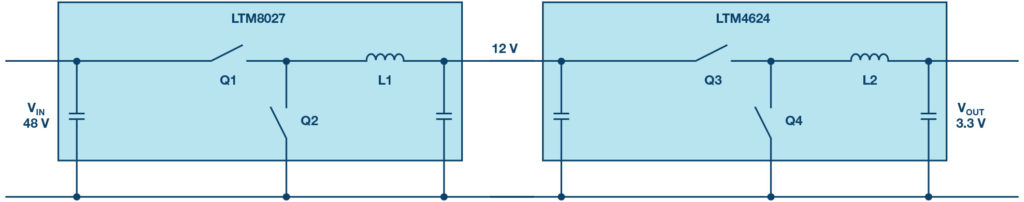## Intermediate voltage for increased power conversion efficiency

Series

By Frederik Dostal, power management technical expert, Analog Devices

## Question:

How to increase the efficiency of a power converter with high voltage input and low voltage output?

There are different solutions to convert a high input voltage to a low output voltage, such as, for example, 48V down to 3.3V. Typically, this is required in server applications for the information technology and telecommunications markets.

If a step-down converter (buck) is used for this single conversion step (Figure 1), there’s the problem of small duty cycles. Typically, duty cycle is defined as the relationship between the on-time and the off-time of the main switch.

However, a buck converter’s duty cycle is defined by the following equation:

With input of 48V and output of 3.3V, the duty cycle is approximately 7%. This means that at a switching frequency of 1MHz (1000ns per switching period), the Q1 switch is turned on for only 70ns. Then, the Q1 switch is turned off for 930ns and Q2 is turned on.

For such a circuit, a switching regulator must be chosen that allows for a minimum on-time of 70ns or less. However, this brings another challenge. Usually, the very high power conversion efficiency of a buck regulator is reduced when operating at short duty cycles, because there’s only a very short time available to store energy in the inductor.

The inductor must provide power for a long period during the off-time, which leads to high peak currents in the circuit. To lower these currents, the inductance of L1 needs to be relatively large, because during the on-time a large voltage difference is applied across L1.

In the example we see about 44.7V across the inductor during the on-time, 48V on the switch-node side and 3.3V on the output side. The inductor current is calculated by:

If there is a high voltage across the inductor, the current rises during a fixed time period and inductance much more than if there was a low voltage across the inductor. To reduce inductor peak currents, a higher inductance value needs to be selected, which then adds to the power losses. Under these voltage conditions, an efficient LTM8027 µModule regulator from Analog Devices achieves power efficiency of 80% at 4A output current.

Today, a very common and more efficient way to increase power efficiency is by generating an intermediate voltage. A cascaded setup with two highly efficient step-down (buck) regulators is shown in Figure 2. In the first step, the 48V is converted to 12V, then to 3.3V in a second conversion step. The LTM8027 µModule regulator has a total conversion efficiency of over 92% when going from 48V to 12V.Figure 2: Voltage conversion from 48V down to 3.3V in two steps, including 12V intermediate voltage

The second conversion step from 12V to 3.3V, performed by an LTM4624, has a conversion efficiency of 90%, yielding a total power conversion efficiency of 83% – 3% higher than the direct conversion in Figure 1. This can be quite surprising since all the power on the 3.3V output needed to run through two individual switching regulator circuits. The efficiency of the circuit in Figure 1 is lower due to the short duty-cycle and the resulting high inductor peak currents.

## Other Aspects

When comparing single step-down architectures with intermediate-bus architectures, there are other aspects to consider besides power efficiency. However, this article is only intended to look at the important aspects of power conversion efficiency.

One other solution to this basic problem is the new LTC7821, a hybrid step-down controller. It combines charge-pump action with step-down buck regulation, enabling the duty cycle to be 2 × VIN/VOUT so that very high step-down ratios can be achieved at very high power-conversion efficiencies.

Generating intermediate voltage can be a quite useful way to increase the total conversion efficiency of a specific power supply. A lot of development goes into increasing the conversion efficiency in Figure 1 with such short duty cycles.

For example, very fast GaN switches can be used, which reduce the switching losses and, as a result, increase the power conversion efficiency. However, such solutions are currently more expensive than cascaded solutions, such as that shown in Figure 2.